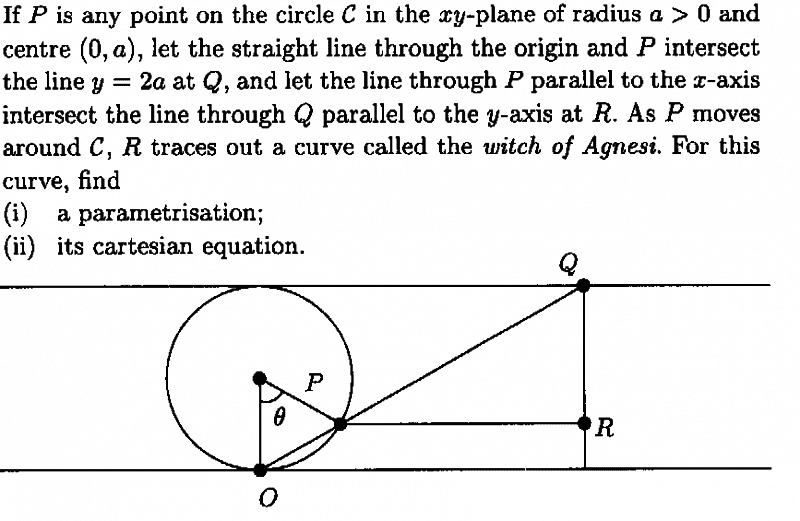# Parametrization of Witch of Agnesi

## Homework StatementThe question is completely described in the photo.

## Homework Equations

Trigonometric translation properties

## The Attempt at a Solution

The problem is in two dimensions, so I'm ignoring the z coordinates. For a circle centered at (0,a), the position vector of P is ##(a## ##sin(θ),a-a## ##cos(θ))## (by taking into consideration what theta is in this problem) since ##a## ##cos(\frac{3π}{2}+θ)=a## ##sin(θ)## and ##a+a## ##sin(\frac{3π}{2}+θ)=a-a## ##cos(θ)## .
Therefore, the y coordinates of R should be ##a-a## ##cos(θ)## . R's x coordinate equals to the x coordinate of Q, which is given by ##x=\frac{2a}{m}## , where ##m=\frac{1-cos(θ)}{sin(θ)}## .
So my answer is ##γ(θ) = (\frac{2asin(θ)}{1-cos(θ)},a-cos(θ))##. However, the correct answer is ##γ(θ) = (2a## ##cot(θ), a(1-cos(2θ))##. Where did I go wrong?